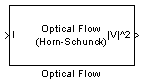# Optical Flow

Estimate object velocities

• Library:
• Computer Vision Toolbox / Analysis & Enhancement

•## Description

The Optical Flow block estimates the direction and speed of object motion between two images or between one video frame to another frame using either the Horn-Schunck or the Lucas-Kanade method.

## Ports

### Input

expand all

Image or video frame, specified as a scalar, vector, or matrix. If the Compute optical flow between parameter is set to `Two images`, the name of this port changes to I1.

Data Types: `single` | `double` | `int8` | `int16` | `int32` | `int64` | `uint8` | `uint16` | `uint32` | `uint64` | `fixed point`

Image or video frame, specified as a scalar, vector, or matrix.

Data Types: `single` | `double` | `int8` | `int16` | `int32` | `int64` | `uint8` | `uint16` | `uint32` | `uint64` | `fixed point`

### Output

expand all

Velocity magnitudes, returned as a scalar, vector, or matrix.

Data Types: `single` | `double` | `int8` | `int16` | `int32` | `int64` | `uint8` | `uint16` | `uint32` | `uint64` | `fixed point`

Velocity components in complex form, specified as a scalar, vector, or matrix.

#### Dependencies

To enable this port, set the Velocity output parameter to ```Horizontal and vertical components in complex form```.

Data Types: `single` | `double` | `int8` | `int16` | `int32` | `int64` | `uint8` | `uint16` | `uint32` | `uint64` | `fixed point`

## Parameters

expand all

Main Tab

Select the method to use to calculate the optical flow. Options include `Horn-Schunck` or `Lucas-Kanade`.

Select how to compute the optical flow. Select `Two images` to compute the optical flow between two images. Select ```Current frame and N-th frame back``` to compute the optical flow between two video frames that are N frames apart.

#### Dependencies

To enable this parameter, set the:

• Method parameter to `Horn-Schunck`

• Method parameter to `Lucas-Kanade` and the Temporal gradient filter to ```Difference filter [-1 1]```

Enter a scalar value that represents the number of frames between the reference frame and the current frame.

#### Dependencies

To enable this parameter, set the Compute optical flow between parameter to ```Current frame and N-th frame back```.

Specify the smoothness factor. Enter a large positive scalar value for high relative motion between the two images or video frames. Enter a small positive scalar value for low relative motion.

#### Dependencies

To enable this parameter, set the Method parameter to `Horn-Schunck`.

Specify the method to control when the block's iterative solution process stops. If you want the process to stop when the velocity difference is below a certain threshold value, select `When velocity difference falls below threshold`. If you want the process to stop after a certain number of iterations, choose `When maximum number of iterations is reached`. You can also select `Whichever comes first`.

#### Dependencies

To enable this parameter, set the Method parameter to `Horn-Schunck`.

Specify the maximum number of iterations for the block to perform.

#### Dependencies

To enable this parameter, set the Method parameter to `Horn-Schunck` and the Stop iterative solution parameter to ```When maximum number of iterations is reached``` or `Whichever comes first`.

Specify how to output an optical flow. If you select `Magnitude-squared`, the block outputs an optical flow matrix where each element is in the form ${u}^{2}+{v}^{2}$. If you select ```Horizontal and vertical components in complex form```, the block outputs the optical flow matrix where each element is in the form $u+jv$.

Specify whether the block solves for u and v using a difference filter or a derivative of a Gaussian filter.

#### Dependencies

To enable this parameter, set the Method parameter to `Lucas-Kanade`.

Specify the number of input frames to buffer for smoothing. Use this parameter for temporal filter characteristics such as the standard deviation and number of filter coefficients.

#### Dependencies

To enable this parameter, set the Temporal gradient filter parameter to `Derivative of Gaussian`.

Specify the standard deviation for the image smoothing filter.

#### Dependencies

To enable this parameter, set the Temporal gradient filter parameter to `Derivative of Gaussian`.

Specify the standard deviation for the gradient smoothing filter.

#### Dependencies

To enable this parameter, set the Temporal gradient filter parameter to `Derivative of Gaussian`.

Select this parameter to set the motion vector to zero when the optical flow constraint equation is ill-conditioned.

#### Dependencies

To enable this parameter, set the Temporal gradient filter parameter to `Derivative of Gaussian`.

Select this parameter to output the image that corresponds to the motion vector outputted by the block.

#### Dependencies

To enable this parameter, set the Temporal gradient filter parameter to `Derivative of Gaussian`.

Specify a scalar value that determines the motion threshold between each image or video frame. The higher the number, the less small movements impact the optical flow calculation.

#### Dependencies

To enable this parameter, set the Method parameter to `Lucas-Kanade`.

Data Types Tab

For details on the fixed-point block parameters, see Specify Fixed-Point Attributes for Blocks.

## Block Characteristics

 Data Types `double` | `single` Multidimensional Signals `no` Variable-Size Signals `no`

expand all

## Version History

Introduced before R2006a